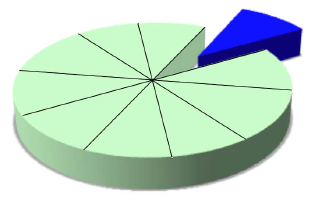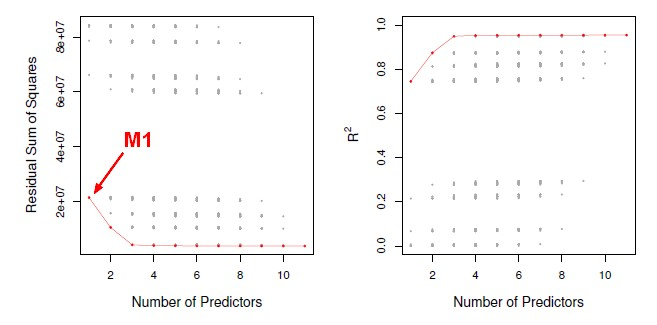# R - K-fold cross-validation (with Leave-one-out)

Cross-validation in R.

## Leave-one-out

### cv.glm

Each time, Leave-one-out cross-validation (LOOV) leaves out one observation, produces a fit on all the other data, and then makes a prediction at the x value for that observation that you lift out.

Leave-one-out cross-validation puts the model repeatedly n times, if there's n observations.

require(boot)
?cv.glm
glm.fit=glm(response~predictor, data=myData)

#LOOCV
cv.glm(myData,glm.fit)$delta    24.23151 24.23114   delta is the cross-validated prediction error where: • The first number is the raw leave-one-out, or lieu cross-validation result. • The second one is a bias-corrected version of it. The bias correction has to do with the fact that the data set that we train it on is slightly smaller than the one that we actually would like to get the error for, which is the full data set of size n. It turns out that has more of an effect for k-fold cross-validation. cv.glm does the computation by brute force by refitting the model all the N times and is then slow. It doesn't exploit the nice simple below LOOCV formula. The reason cv.glm doesn't use that formula is that it's also set up to work on logistic regressions and other models, and there the shortcut doesn't work. ### Shortcut Formula The magic formula for least square regression: $$CV_{(n)} = \frac{1}{n} \sum^n_{i=1} \left ( \frac{ \href{residual}{y_i - \hat{y}_i} }{ 1-h_i} \right ) ^2$$ So: • The error would be the ordinary residuals if we didn't leave the observations out. • The numerator comes from the least squares fit but we divide them by $(1- h_i)^2$ The $h_i$ is the diagonal element of the hat matrix. The hat matrix is the operator matrix that produces the least squares fit. This is also known as the self influence. It's a measure of how much observation i contributes to it's own fit. The values of $h_i$ vary between 0 and 1. If $h_i$ is close to 1 (ie observation i contributes really a lot to its own fit), $1- h_i$ is small. And that will inflate the residual. Function that represents the above formula: loocv=function(fit){ h=lm.influence(fit)$h
mean((residuals(fit)/(1-h))^2)
}


where:

• the function ln.influence is a post-processor for ln fit. It'll extract the element h from that and gives you the diagonal elements $h_i$

Example of use: measure of errors for polynomials fit with different degrees

# A vector for collecting the errors.
cv.error=vector(mode="numeric",length=5)
# The polynomial degree
degree=1:5
# A fit for each degree
for(d in degree){
glm.fit=glm(response~poly(predictor,d), data=myDataFrame)
cv.error[d]=loocv(glm.fit)
}
# The plot of the errors
plot(degree,cv.error,type="b")


## 10-fold cross-validation

With 10-fold cross-validation, there is less work to perform as you divide the data up into 10 pieces, used the 1/10 has a test set and the 9/10 as a training set. So for 10-fall cross-validation, you have to fit the model 10 times not N times, as loocv

## 10-fold CV
# A vector for collecting the errors.
cv.error10=rep(0,5)
# The polynomial degree
degree=1:5
# A fit for each degree
for(d in degree){
glm.fit=glm(response~poly(predictor,d), data=myDataFrame)
cv.error10[d]=cv.glm(myDataFrame,glm.fit,K=10)\$delta
}
lines(degree,cv.error10,type="b",col="red")


In general, 10-fold cross-validation is favoured for computing errors. It tends to be a more stable measure than leave-one-out cross-validation. And for the most time, it's cheaper to compute.

Discover More(Statistics|Data Mining) - (K-Fold) Cross-validation (rotation estimation)

Cross-validation, sometimes called rotation estimation is a resampling validation technique for assessing how the results of a statistical analysis will generalize to an independent new data set. This...Statistics - Model Selection

Model selection is the task of selecting a statistical model from a set of candidate models through the use of criteria's Dimension reduction procedures generates and returns a sequence of possible...